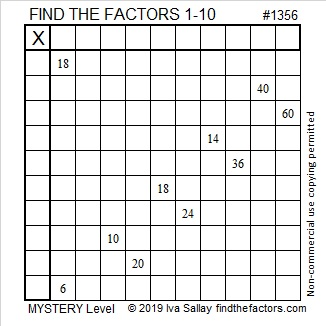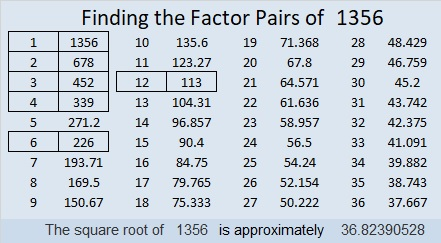# 1356 Mystery

The clues that appear in this puzzle are all you need to find all the factors. Seriously. Some mysteries are easier to solve than others. Give this one a try!Print the puzzles or type the solution in this excel file: 10 Factors 1347-1356

Here is some information about the puzzle number, 1356:

• 1356 is a composite number.
• Prime factorization: 1356 = 2 × 2 × 3 × 113, which can be written 1356 = 2² × 3 × 113
• 1356 has at least one exponent greater than 1 in its prime factorization so √1356 can be simplified. Taking the factor pair from the factor pair table below with the largest square number factor, we get √1356 = (√4)(√339) = 2√339
• The exponents in the prime factorization are 2, 1, and 1. Adding one to each exponent and multiplying we get (2 + 1)(1 + 1)(1 + 1) = 3 × 2 × 2 = 12. Therefore 1356 has exactly 12 factors.
• The factors of 1356 are outlined with their factor pair partners in the graphic below.1356 is the hypotenuse of a Pythagorean triple:
180-1344-1356 which is 12 times (15-112-113)

This site uses Akismet to reduce spam. Learn how your comment data is processed.